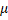# A smooth ball is dropped from heighton a smooth incline as shown in Fig. After collision, the velocity of the ball is directed horizontallyFind the coefficient of restitution a)b)c)d)## Question ID - 101129 :- A smooth ball is dropped from heighton a smooth incline as shown in Fig. After collision, the velocity of the ball is directed horizontallyFind the coefficient of restitution a)b)c)d)3537

(c)(i)From Eqs. (i) and (ii),Next Question :
 Two different light sources of light A and B have wavelength 0.7m and 0.3m respectively. Then which of the following statement is true? (a) A has greater energy than B (b) B has greater energy than A (c) Both has equal energy (d) None of these